# NEET Phy COM (Study Notes)

CONTENTS

Centre of mass of a two-particle system; Centre of mass of a rigid body; Velocity of Centre of Mass; Acceleration of Centre of Mass; Some Important Points

CENTRE OF MASS

It is point in a system which moves as if whole mass of the system is concentrated at the point and all external forces are acting on it. Its position is given by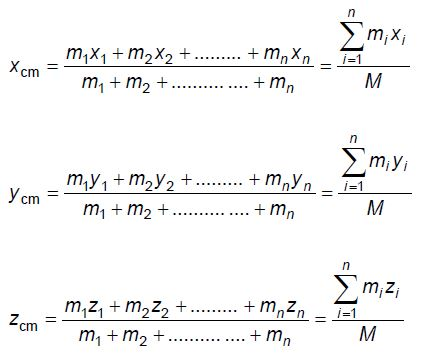CENTRE OF MASS OF A RIGID BODY

Mathematically position coordinates of the centre of mass of rigid body are given  byVELOCITY AND ACCELERATION OF CENTRE OF MASS

Velocity of Centre of Mass:

The instantaneous velocity of centre of mass is given by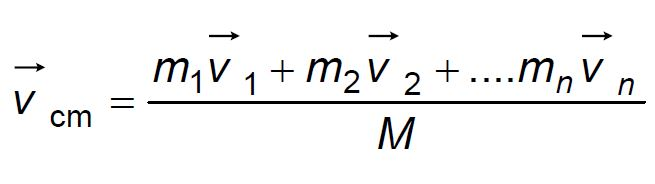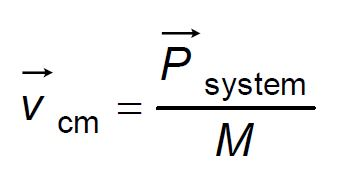Acceleration of Centre of Mass:Some Important Points

(1) The centre of mass of a system of particles lies in the region where majority of mass of the system lies.

(2) The centre of mass of a body may lie outside the body or on the body. e.g., The centre of mass of a ring lies at its centre not on its body.

(3) For a symmetrical body of uniform density, the centre of mass lies at its geometrical centre.

(4) For a two particle system, the centre of mass lies on the line joining them and closer to the greater mass. The position co-ordinates of the centre of mass of a system of two masses as shown in the figure.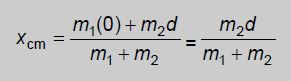(5) The centre of mass is a point such that the mass of the system M multiplied by the acceleration of the centre of mass of the system gives the resultant of all forces acting on the system.

(6) The centre of mass of a system of two particles lies in between them on the line  joining the particles.

(7) If we take the centre of mass as the origin, then the sum of the moments of the masses of the system is zero.

(8) During translatory motion, the position of the centre of mass changes with time.

(9) In the absence of an external force, the velocity of the centre of mass of a body remains constant.

(10) The location of the centre of mass depends on the shape and nature of distribution of mass of the body.

Example :

The position co-ordinates of the centre of mass of a system of three particles of equal masses which are placed on the vertices of an equilateral triangle of side a are calculated as shown.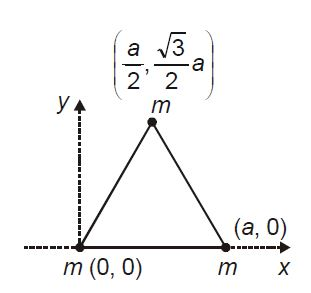Co-ordinates of the centre of mass of the system are (a/2, a/2√3)

Announcements

Join our Online JEE Test Series for 499/- Only (Web + App) for 1 Year

Join our Online NEET Test Series for 499/- Only for 1 Year